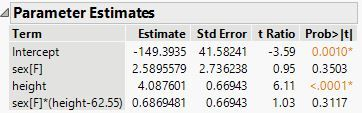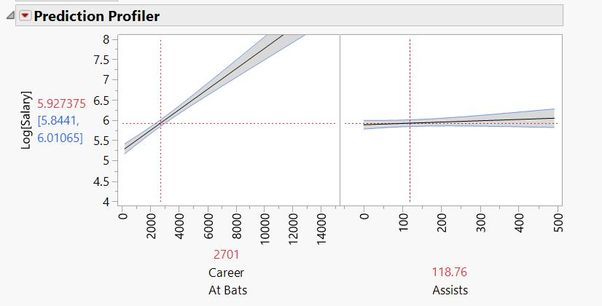Choose Language Hide Translation Bar
Highlighted

## Interactions in OLS regression and simple slopes.

Hey everyone,

I am currently trying to perform an OLS (linear) regression model with an interaction effect (to test for moderation). For example, let's say I wish to predict a continuous variable Y using two continuous variables X and M and their interaction X*M.

The result of the effect of the interaction term is significant. However, I do not know how to ask JMP to give the data for the simple slopes.
Specifically, how do I get the betas and their significance for the effect of X an Y at the different levels of M? (usually -1sd, mean, 1sd for continuous variable or if the moderator is binary than at both levels of the moderator).

I know how to get the plots (Fit model ... Full factorial etc.), but it's not enough, I need the actual simple effects for the slopes, SE and significance.

Will appreciate any help on the matter.

11 REPLIES 11
Highlighted

## Re: Interactions in OLS regression and simple slopes.

You must be using the Fit Least Squares platform to include a term for an interaction effect in the linear predictor. The Parameter Estimates report provides the estimate (slope), standard errors, and t-ratios. For example:So the effect (slope) of height for sex=F is 4.087601 + 0.6869481 and for sex=M it is 4.087601 - 0.6869481.

Learn it once, use it forever!
Highlighted

## Re: Interactions in OLS regression and simple slopes.

That different than the way I understand breaking down interactions work.

http://web.pdx.edu/~newsomj/mvclass/ho_simple%20slopes.pdf

this procedure is very easily done via the Process macro for SPSS or via a simple R script.

I just really hope there's a solution for this via JMP...

Highlighted

## Re: Interactions in OLS regression and simple slopes.

JMP emphasizes visualization to understand the nature of the 'simple slopes,' or the profile of the effect of one factor while holding another factor at a given level.

Click the red triangle next to Fit Least Squares and select Factor Profiling > Profiler. This interactive plot is based on the whole model, but shows the profile of each factor individually. You do not need the conditional slope itself because JMP plots the line, curve, or profile for you. This same visualization is available for all of the models in JMP.

Learn it once, use it forever!
Highlighted

## Re: Interactions in OLS regression and simple slopes.

The document you attached describing simple slopes is exactly what @markbailey  described. The only difference is that Mark stated the parameters for a categorical Z in your case (Male (-1) or Female (+1)).

To see this, from your attachment, the model was Y = -2.3X + -0.5Z + 1.6XZ + 3.0. They chose a value of 3.5 for Z. Plugging that in for Z yields Y = (3.0 + 3.5*(-0.5)) + (-2.3 +1.6*3.5)X. The -2.3 + 1.6*3.5 = 3.3 X which exactly matches their result. Mark suggested the same thing, but used -1 and +1 because that is how the male and female values are coded in JMP. The categorical factor makes the "simple slopes" easier to find.

A JMP script could be written to do everything outlined in your attachment (similar to what was done for SPSS or R), but I am not sure that is needed. In all fairness, I would need to explore this further to be certain as I have not looked in detail at the simple slopes document. Anyhow, here are some things to look at to see if such a script would be necessary.

The prediction profiler is your friend! Dragging the value of Z in the profiler will show you the various simple slopes for X. This is a powerful way to see the X slopes for ANY setting of Z rather than just a select few. The prediction profiler also provides error bands to assess the statistical significance of that X slope. If the bands could contain a horizontal line, not significant.

Add the Interaction Profiler from the red popup triangle. That will show the slope for the lowest Z setting AND the highest Z setting.

Finally, your document has the sentence: "The usual recommendation is to center the predictor variables by subtracting the mean of each and then computing the interaction term." When your Z is continuous, JMP will do this automatically. Thus, JMP is following the usual recommendation which allows a straight-forward way to implement the simple slopes idea.

I would suggest taking a worked out dataset with all of the simple slope calculations and run it through JMP to see where the worked out calculations match the JMP output.

Dan Obermiller
Highlighted

## Re: Interactions in OLS regression and simple slopes.

First, thank you both for your help, it is much appreciated.

I do need the simple slopes as I must report them in my study's results section - I can't just describe it without including the actual values as is accepted (the b(SE), p value) for -1sd, mean and +1sd.

thus, the question is, is there a way to get those in JMP?

Once again, thank you very much for your help!

Highlighted

## Re: Interactions in OLS regression and simple slopes.

I see no way to get those items "automatically" with JMP. You get the information needed to make the decision, but not actual values to report. So, a JMP script would need to be created to do it.

As your document says: "... most software programs have not created prepackaged procedures for plotting or testing simple slopes."

Look in the JMP Wish List to see if someone has added this feature to the list. If so, just kudo that entry so the developers know the support for that feature. If you don't find it there, add it so that it can be considered for a future release of JMP.

Dan Obermiller
Highlighted

Thank you.

Highlighted

## Re: Interactions in OLS regression and simple slopes.

Dear @Dan_Obermiller, I encountered similar problems with the Simple Slope Analysis and your answer proved to be very helpful. Can you explain more about how to use the error bands to find the statistical significance of the X slope?

(For example, how to show the Error Bands since I can only find the Interval Confidence in the Prediction Profiler? Can I find the exact significance level or just know that the Simple Slope is significant)

I can already calculate the β for the Simple Slope at -1 SD, +1 SD and Mean. The only thing left is calculating the Signiificance Level.

If it can be of any help, here is my data.

Highlighted

## Re: Interactions in OLS regression and simple slopes.

Unfortunately I do not have the time right now to build the tests on the simple slopes. Perhaps someone else can help with that. One thing that I did notice on your attached picture is that your prediction profiler does not have the confidence intervals turned on. If you click on the red triangle for the profiler, choose Confidence Intervals. You should see bands show up like this picture:Although this is not your data, notice the picture on the left. There is a horizontal line that is NOT within the shaded confidence interval. That variable (Career At Bats) is significant. The shaded confidence interval on the right completely contains the horizontal mean line. That variable is NOT significant. These are 95% confidence intervals and would be equivalent to conducting the test at the 5% significance level.

Dan Obermiller
Article Labels

There are no labels assigned to this post.